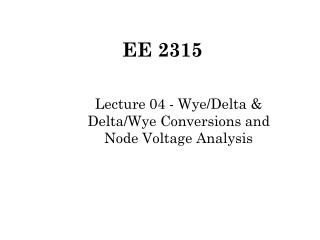# EE 2315 - PowerPoint PPT PresentationDownload PresentationEE 2315

EE 2315
Download Presentation## EE 2315

- - - - - - - - - - - - - - - - - - - - - - - - - - - E N D - - - - - - - - - - - - - - - - - - - - - - - - - - -
##### Presentation Transcript

1. EE 2315 Lecture 04 - Wye/Delta & Delta/Wye Conversions and Node Voltage Analysis

2. Wye/Delta Conversion (1/2) Wye Delta

3. Wye/Delta Conversion (2/2)

4. Delta/Wye Conversion

5. Effects of D-Y & Y-D Conversions • D-Y Conversion eliminates a loop but adds a node. • Y-D Conversion eliminates a node but adds a loop.

6. Y-D Conversion Example Find Rab Several Methods: We choose to eliminate node c, then simplify.

7. Y-D Conversion Calculations

8. New Circuit

9. Further Simplifications

10. Introduction to Node Voltage Method • More direct than branch equations • Fewer equations to solve • Choose one node as reference • Express all variables in terms of node voltages • Solution is set of node voltages w.r.t. chosen reference node • Solution completely defines the circuit

11. Node Voltage Example 1 (1/4) Apply KCL at Node 1: Use Ohm’s Law:

12. Node Voltage Example 1 (2/4) Apply KCL at Node 2: Use Ohm’s Law:

13. Node Voltage Example 1 (3/4) KCL for Node 1: KCL for Node 2:

14. Node Voltage Example 1 (4/4)

15. Node Voltage Example 1a (1/2)

16. Node Voltage Example 1a (2/2)

17. Node Voltage Example 2 (1/2) KCL for Node 1: KCL for Node 2:

18. Node Voltage Example 2 (2/2) Substitute: into KCL equations Node 1: Node 2:

19. Node Voltage Example 2a (1/2) Substituting for ix:

20. Node Voltage Example 2a (2/2)

21. Node Voltage Example 3 (1/2) KCL for Node 1: KCL for Node 2:

22. Node Voltage Example 3 (2/2) Substitute: into KCL equations Node 1: Node 2:

23. Node Voltage Example 3a (1/2) After Substitution:

24. Node Voltage Example 3a (2/2) Substitute and solve:

25. Node Voltage Example 4 (1/3) No KCL equation possible at node 1 or at node 2. KCL can be applied at the supernode.

26. Node Voltage Example 4 (2/3) KCL @ Supernode: Other relationships: and

27. Node Voltage Example 4 (3/3)

28. Node Voltage Example 4a (1/2)

29. Node Voltage Example 4a (2/2) Substitute and solve:

30. Alternative to Supernode (1/2) KCL @ Node 1: KCL @ Node 2:

31. Alternative to Supernode (2/2) Use Ix to get same info as Supernode: